Courses

# Solution of Linear Equations - Matrices and Determinants, Business Mathematics & Statistics B Com Notes | EduRev

## B Com : Solution of Linear Equations - Matrices and Determinants, Business Mathematics & Statistics B Com Notes | EduRev

The document Solution of Linear Equations - Matrices and Determinants, Business Mathematics & Statistics B Com Notes | EduRev is a part of the B Com Course Business Mathematics and Statistics.
All you need of B Com at this link: B Com

SOLUTION OF LINEAR EQUATIONS  unique solution given by X = A-1B. Example 24 Solve using matrices the equations 2x- y = 3, 5x+y = 4. Solution :
The equations can be written in matrix form as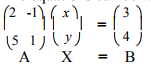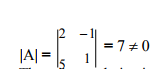∴ The unique solution is given by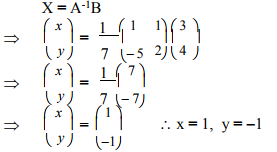Example 25 Solve the equations 2x +8y +5z = 5, x +y +z = -2, x +2y -z = 2 by using matrix method.
Solution : The equations can be written in matrix form as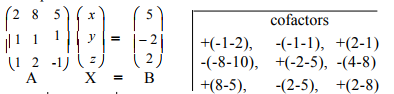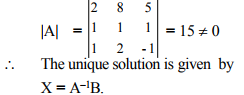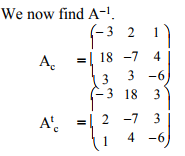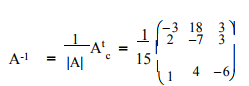Now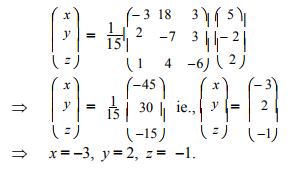Example 26
A woman invested different amounts at 8%, 8 4 % and 9%, all at simple interest. Altogether she invested Rs. 40,000 and earns Rs. 3,455 per year. How much does she have invested at each rate if she has Rs. 4,000 more invested at 9% than at 8%? Solve by using matrices.

Solution :
Let x, y, z be the amounts in Rs. invested at 8%, 8 3 % and 4
9% respectively.
According to the problem,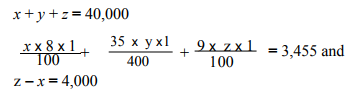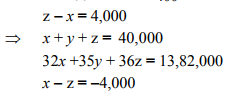The equations can be written in matrix form as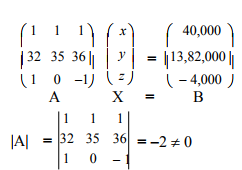∴ The unique solution is given by X = A-1B We now find A-1 .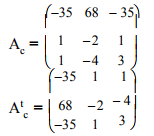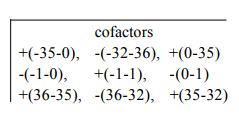Now,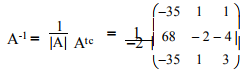Now,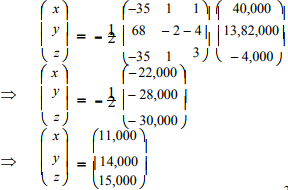Hence the amounts invested at 8%, 8 3 /4% and 9% are 4 Rs. 11,000, Rs. 14,000 and Rs. 15,000 respectively.

Solution by Determinant method (Cramer’s rule) Let the equations be
a1 x + b1 y + c, z = d1 ,
a2 x + b2 y + c2 z = d2 ,
a3 x + b3 y + c3 z = d3 .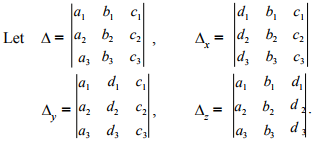When Δ ≠ 0, the unique solution is given by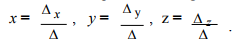Example 27 Solve the equations x +2y +5z = 23, 3x +y +4z = 26, 6x +y +7z = 47 by determinant method.

Solution :
The equations are
x +2y +5z = 23
3x +y +4z = 26
6x +y +7z = 47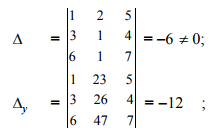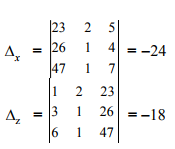By Cramer's rule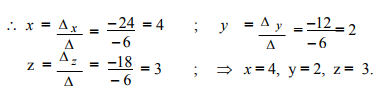Example 28 Solve the equations 2x-3y-1 = 0, 5x +2y -12 = 0 by Cramer’s rule.
Solution :
The equations are 2x - 3y = 1, 5x +2y = 12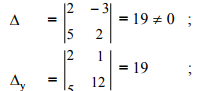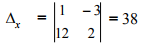By Cramer's rule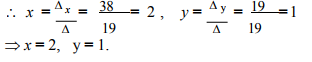Example 29 A salesman hasthe following record ofsales during three months for three items A, B and C which have different rates of commission.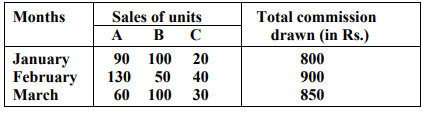Find out the rates of commission on the items A, B and C. Solve by Cramer’s rule.

Solution :
Let x, y and z be the rates of commission in Rs. per unit for A, B and C items respectively.

According to the problem,
90x +100y +20z = 800
130x +50y +40z = 900
60x +100y +30z = 850

Dividing each of the equations by 10 throughout,
9x +10y + 2z = 80
13x + 5y + 4z = 90
6x + 10y + 3z = 85

Now,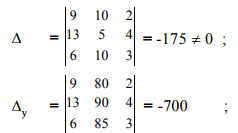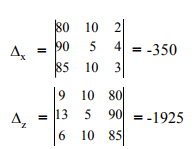By Cramer's rule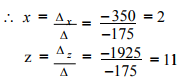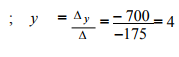Hence the rates of commission for A, B and C are Rs. 2, Rs. 4 and Rs. 11 respectively.

Offer running on EduRev: Apply code STAYHOME200 to get INR 200 off on our premium plan EduRev Infinity!

122 videos|142 docs

,

,

,

,

,

,

,

,

,

,

,

,

,

,

,

,

,

,

,

,

,

,

,

,

;